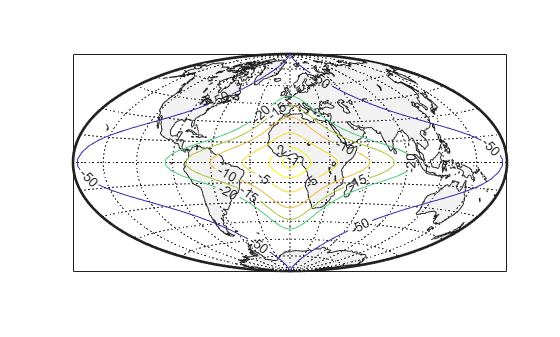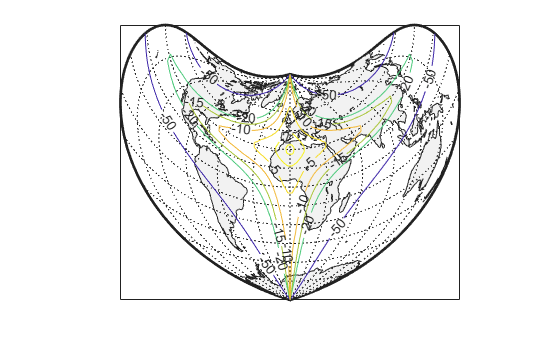# Visualize Projection Distortions Using Isolines

This example shows how to visualize map projection distortions using isolines (contour lines). Since distortions are rather orderly and vary continuously, they are well-suited for isolines. The `mdistort` function can plot variations in angles, areas, maximum and minimum scale, and scale along parallels and meridians, in units of percent deviation (except for angles for which degrees are used).

Create a Hammer projection map axes in normal aspect and plot a graticule and frame.

```figure axesm('MapProjection','hammer','Grid','on','Frame','on')```Load the coast data set and plot it as green patches.

```load coastlines patchm(coastlat,coastlon,'g')```Plot contours of minimum-to-maximum scale ratios, using `mdistort` . Notice that the region of minimum distortion is centered around (0,0).

`mdistort('scaleratio')`Repeat this diagram with a Bonne projection in a new figure window. Notice that the region of minimum distortion is centered around (30,0) which is where the single standard parallel is. You can toggle the isolines by typing `mdistort` or `mdistort off` .

```figure axesm('MapProjection','bonne','Grid','on','Frame','on') patchm(coastlat,coastlon,'g') mdistort('scaleratio')```## SupportGet trial now